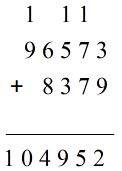Courses
Courses for Kids
Free study material
Free LIVE classes
More# What would be the digit in Ten-thousand’s place in the sum of 96,573 and 8,379?Verified
328.5k+ views
Hint: In any number if we are starting from the rightmost digit, first comes Ones place then Tens, Hundred, Thousand, Ten-thousand, Hundred- thousand and so on. This concept is included in the number system. Add the given numbers and check the position in the final result accordingly.Therefore the required sum obtained = 104,952
Here, 2 is at one’s place.
5 is at ten’s place.
9 is in hundreds place.
4 is at thousand’s place.
0 is at ten- thousands place.
1 is in a hundred thousands place.
Therefore, 0 is the digit in ten thousand’s place.

Note: Concept of number system must be clear. We should know how to carry a digit in a sum. The sum must be accurate and we must follow the rules of the number system. If the sum is wrong, we will get wrong digits in every place.
Last updated date: 02nd Jun 2023
Total views: 328.5k
Views today: 8.84k